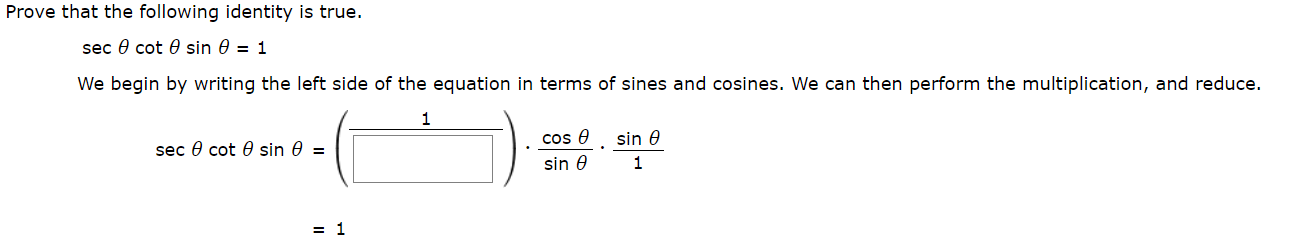# Prove that the following identity is true.sec e cot e sin 0 = 1We begin by writing the left side of the equation in terms of sines and cosines. We can then perform the multiplication, and reduce.1sin ecos esec 0 cot 0 sin e =1sin e= 1

Question
1 views

photo attachedhelp_outlineImage TranscriptioncloseProve that the following identity is true. sec e cot e sin 0 = 1 We begin by writing the left side of the equation in terms of sines and cosines. We can then perform the multiplication, and reduce. 1 sin e cos e sec 0 cot 0 sin e = 1 sin e = 1 fullscreen
check_circle

Step 1

From reciprocal property of trigonometry...

### Want to see the full answer?

See Solution

#### Want to see this answer and more?

Solutions are written by subject experts who are available 24/7. Questions are typically answered within 1 hour.*

See Solution
*Response times may vary by subject and question.
Tagged in

### Trigonometry